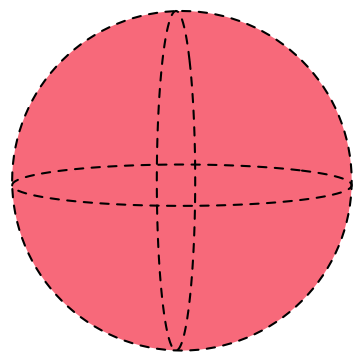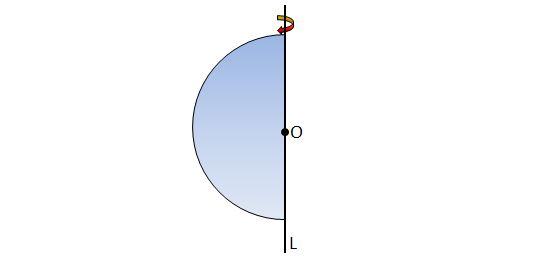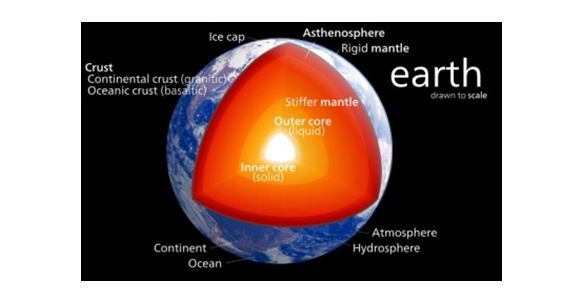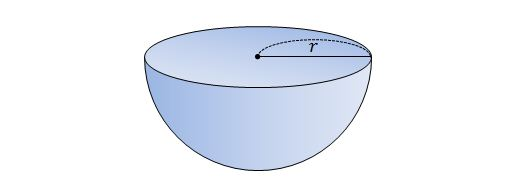Geometry

Volume - SphereIf a sphere has radius 3, what is its volume?What is the volume of the solid figure obtained by rotating a semicircle with radius $6$ around line $L$ containing the diameter of the semicircle (as shown in the above diagram)?

Find the volume of a spherical wedge with $r=7,$ $\theta=\frac{1}{12}\pi.$Calvin is making a model Earth in his science class. The radius of the inner core of the model Earth is $3\text{ cm}$ and the radius of the entire model Earth is $18\text{ cm}.$ How many times larger is the volume of the entire model Earth than the volume of the inner core of the model Earth?

Note: Both the model Earth and its inner core are perfect spheres.If the radius of the hemisphere in the above diagram is $r=11\text{ cm},$ what is the volume of the hemisphere?

×